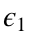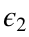# Assignment 6

*** Due date: Start of class in Week 7 ***

## Part 1: Implement the Pure Expected Value Theory model (2 points)

• Follow along with the notes to implement the pure version of Expected Value theory with no noise
• What does pure EV theory do when the risky option is a 25% chance of $19.74? • What does pure EV theory do when the risky option is a 75% chance of$19.97?

• Simulate the choice probabilities for Noisy Expected Value theory when the risky option is a 75% chance of $19.97 ## Part 4: Plot the choice curves for Noisy Expected Value Theory (2 points) • Compute the choice probabilities for Noisy Expected Value Theory when there's a Y% chance of$20, where Y varies from 0.25 to 0.75 in steps of 0.01.
• Plot these choice probabilities as a function of EV_safe - EV_risky to plot the choice curves for NEV theory

## Part 5: Compute the softmax choice curve and compare it with the Noisy Expected Value Theory choice curve (2 points)

• Follow along with the notes to compute the theoretical choice curve from the softmax model
• Plot the theoretical choice curve on the same plot as the simulated choice curve. How well do they compare?

## Part 6: The four parameter "softmax" function (2 Extra Credit Points)

Plot the choice curve for this function ...for the free parameters β (same as before and related to σ), θ (set it to 5 to start with),set to 0.05, andset to 0.1.
By messing around with the parameter values, or thinking carefully about the equation above, what do θ,andcontrol on the choice curve? What cognitive processes could these parameters be capturing?